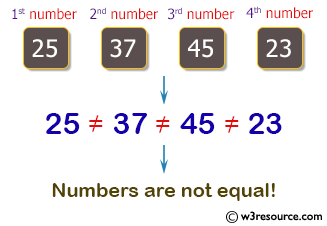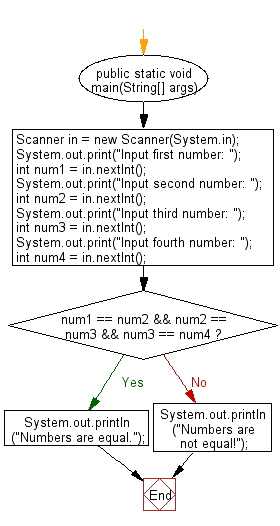﻿ Java programming exercises: Accepts four integer from the user and prints equal - w3resource# Java Exercises: Accepts four integer from the user and prints equal

## Java Basic: Exercise-152 with Solution

Write a Java program that accepts four integer from the user and prints equal if all four are equal, and not equal otherwise.

Pictorial Presentation:Sample Solution:

Java Code:

``````import java.util.Scanner;
public class Solution {
public static void main(String[] args) {
Scanner in = new Scanner(System.in);
System.out.print("Input first number: ");
int num1 = in.nextInt();
System.out.print("Input second number: ");
int num2 = in.nextInt();
System.out.print("Input third number: ");
int num3 = in.nextInt();
System.out.print("Input fourth number: ");
int num4 = in.nextInt();

if (num1 == num2 && num2 == num3 && num3 == num4)
{
System.out.println("Numbers are equal.");
}
else
{
System.out.println("Numbers are not equal!");
}
}
}

```
```

Sample Output:

```Input first number:  25
Input second number:  37
Input third number:  45
Input fourth number:  23
Numbers are not equal!
```

Flowchart:Java Code Editor:

What is the difficulty level of this exercise?

﻿

## Java: Tips of the Day

Parsing dates:

```import java.io.*;
import java.util.*;
import java.text.*;

String s = "2001/09/23 14:39";

SimpleDateFormat formatter = new SimpleDateFormat ("yyyy/MM/dd H:mm");
Date d = formatter.parse(s, new ParsePosition(0));
```